# 【江南百景图进阶攻略】教你如何日赚五十万铜！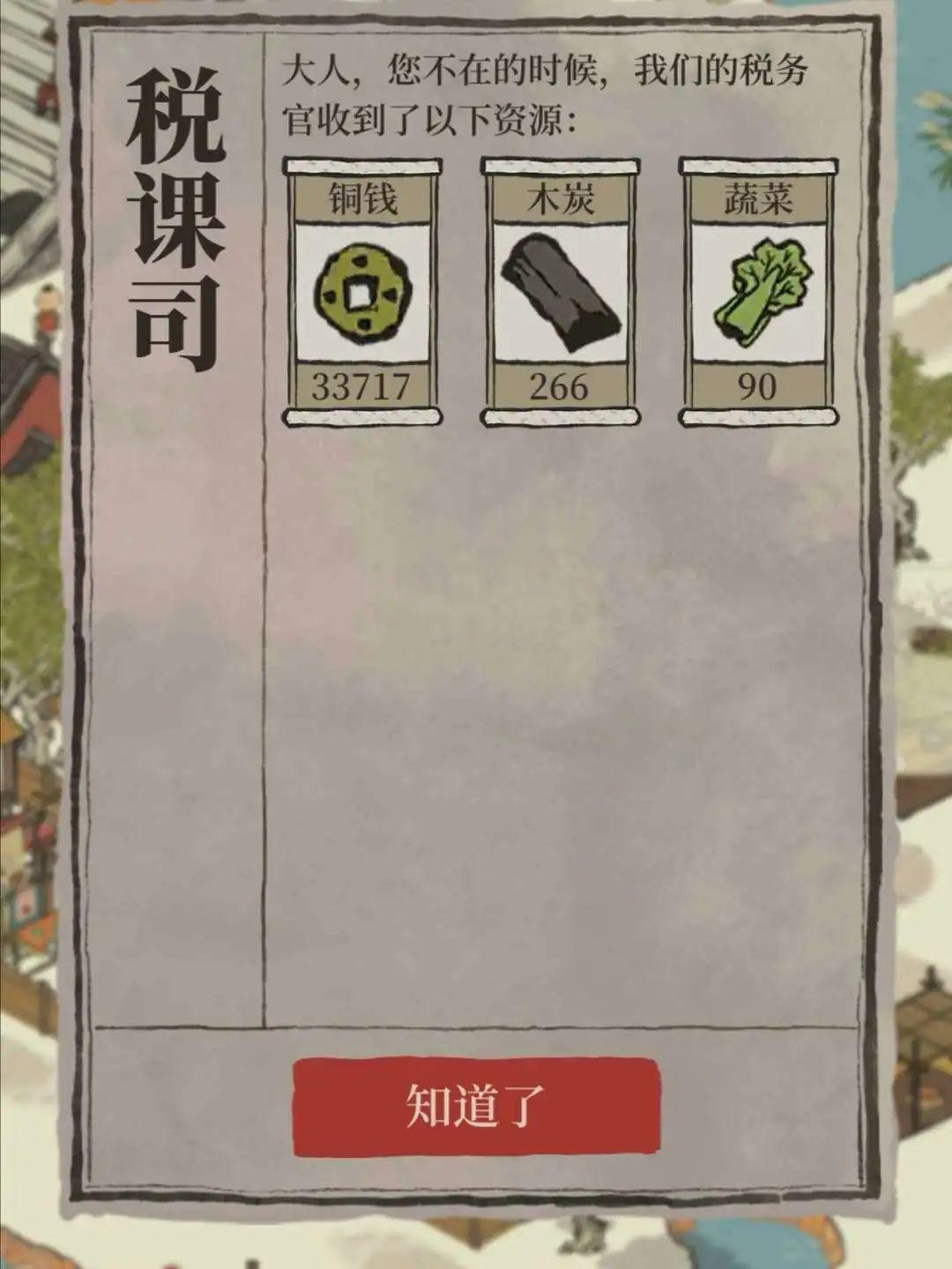16渔具店布局及收益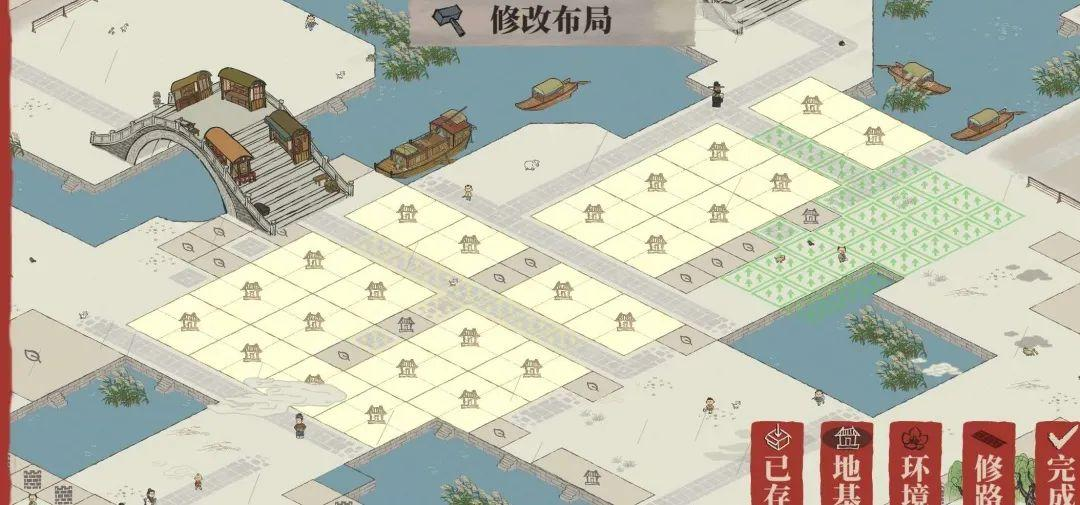16渔具店第三档每轮净收入：320+240-150=410  410*16=6560铜币

12药铺布局及收益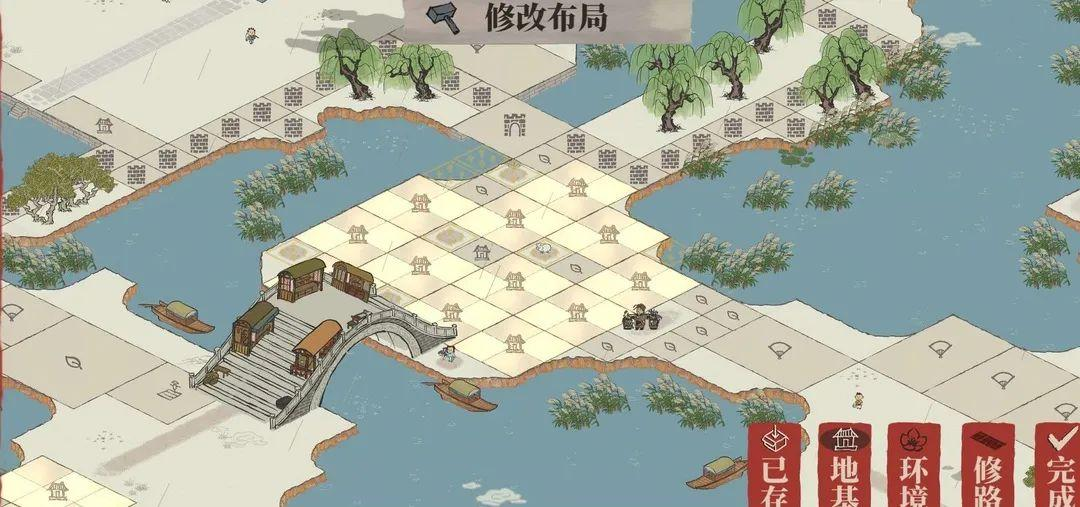12药铺第三档每轮净收入：560+420-250=730   730*12=8760铜币

8寿材店布局及收益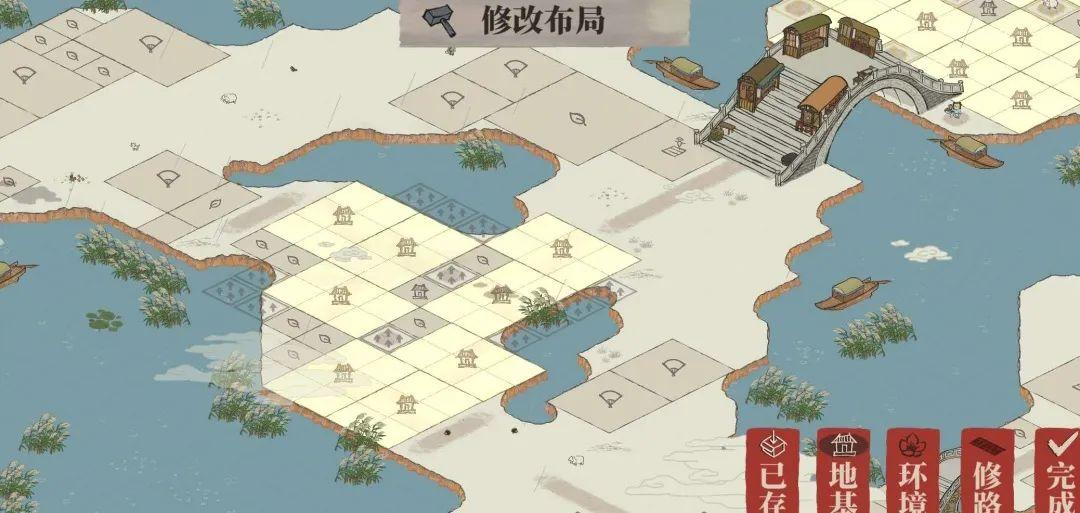8寿材店第三档每轮净收入：600+300-270=630  630*8=5040铜币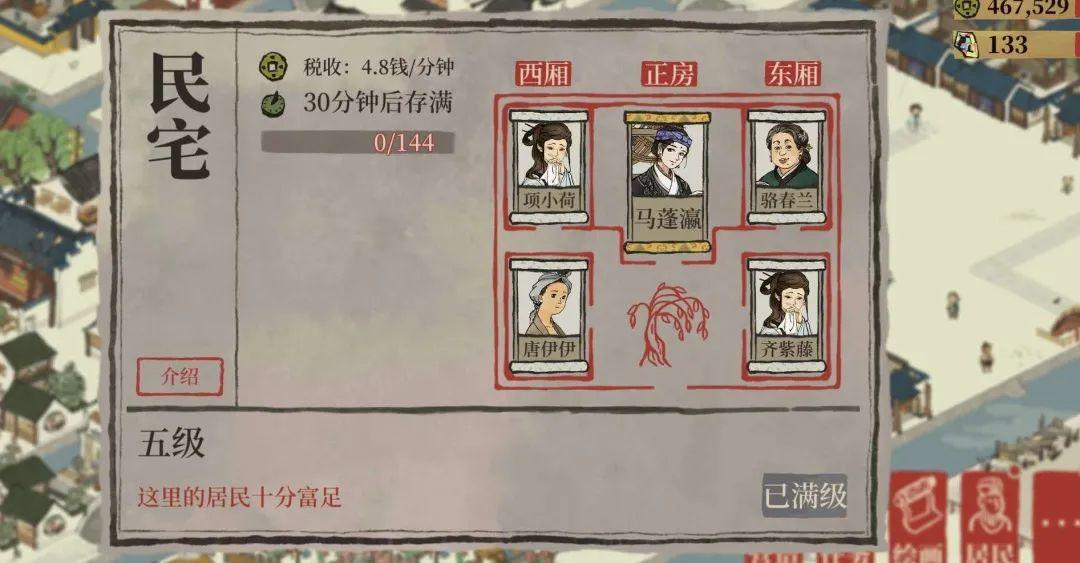19120*10=191200铜币。

16*273*10=43680铜币

32*288*24=221184铜币

191200+43680+221184=456064铜币！